# KS1 Maths Quiz - Year 2 Time - Telling the Time to 5 Minutes (Questions)

This quiz addresses the requirements of the National Curriculum KS1 Maths and Numeracy for children aged 6 and 7 in year 2. Specifically this quiz is aimed at the section dealing with telling the time to 5 minutes.

Once children in Year 2 are familiar with telling the time to the hour, half hour and quarter hour, they begin to recognise how to tell the time to 5 minutes. This may be linked to their work on the five times table, as they link this to counting around the clock in 5s to work out what time it is to five minutes.

To see a larger image, click on the picture.
1. What time will it be in 5 minutes?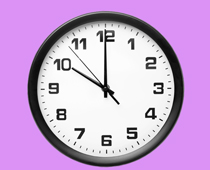[ ] One minute past ten [ ] Five past five [ ] Ten past five [ ] Five past ten
2. What time will it be in 20 minutes?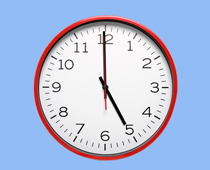[ ] Four minutes past five [ ] Twenty past five [ ] Five past four [ ] Five minutes to four
3. What time is this?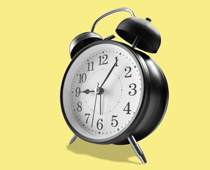[ ] One minute past nine [ ] Five past nine [ ] Nine minutes to one [ ] Five to nine
4. What time is this?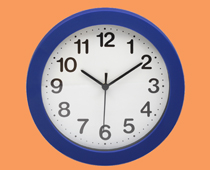[ ] Ten to ten [ ] Two minutes past ten [ ] Ten to two [ ] Ten past ten
5. If the number 12 is at the top, how many minutes past the hour does this watch show?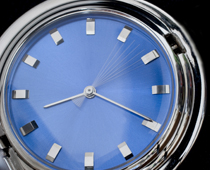[ ] 4 [ ] 20 [ ] 5 [ ] 10
6. How many minutes past the hour does this clock show?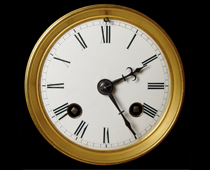[ ] 20 [ ] 15 [ ] 5 [ ] 25
7. How many minutes to 12?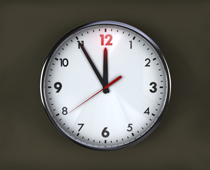[ ] 10 [ ] 5 [ ] 11 [ ] 12
8. How many minutes to 7?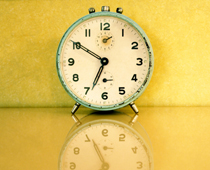[ ] 7 [ ] 20 [ ] 10 [ ] 50
9. What time is this?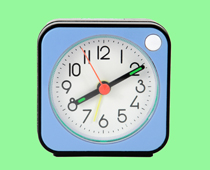[ ] Twenty to two [ ] Two minutes past eight [ ] Eight minutes past ten [ ] Ten past eight
10. What time is this?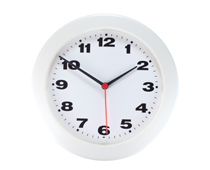[ ] Ten to two [ ] Two minutes past ten [ ] Two minutes to ten [ ] Half past tenKS1 Maths Quiz - Year 2 Time - Telling the Time to 5 Minutes (Answers)
1. What time will it be in 5 minutes?[ ] One minute past ten [ ] Five past five [ ] Ten past five [x] Five past ten
In 5 minutes, the minute hand will have moved to the 1, which shows us five past the hour
2. What time will it be in 20 minutes?[ ] Four minutes past five [x] Twenty past five [ ] Five past four [ ] Five minutes to four
In 20 minutes, the minute hand will point to the 4, which shows us twenty past the hour
3. What time is this?[ ] One minute past nine [x] Five past nine [ ] Nine minutes to one [ ] Five to nine
The minute hand has moved on 5 minutes from the 'o'clock' position, so it is 5 past the hour
4. What time is this?[ ] Ten to ten [ ] Two minutes past ten [ ] Ten to two [x] Ten past ten
The minute hand is 10 minutes past the hour
5. If the number 12 is at the top, how many minutes past the hour does this watch show?[ ] 4 [x] 20 [ ] 5 [ ] 10
Each point on the face is 5 minutes and 4 x 5 = 20
6. How many minutes past the hour does this clock show?[ ] 20 [ ] 15 [ ] 5 [x] 25
The minute hand has moved 25 minutes past the 'o'clock' position
7. How many minutes to 12?[ ] 10 [x] 5 [ ] 11 [ ] 12
It will take the minute hand 5 minutes to reach the top, when it will be 12 o'clock
8. How many minutes to 7?[ ] 7 [ ] 20 [x] 10 [ ] 50
It is 10 minutes until the minute hand is back at the top
9. What time is this?[ ] Twenty to two [ ] Two minutes past eight [ ] Eight minutes past ten [x] Ten past eight
The minute hand has travelled 10 minutes past the 'o'clock' so it's ten past eight
10. What time is this?[x] Ten to two [ ] Two minutes past ten [ ] Two minutes to ten [ ] Half past ten
There are ten minutes until it's two o'clock